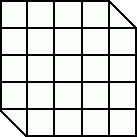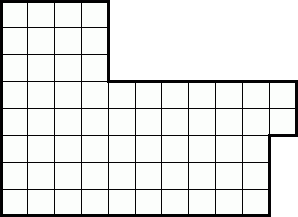LANGUAGE:

# Geometry Puzzles

## 6x4 Rectangle

Your task is to dissect pictures to rearange the pieces into new shapes in the following brain puzzles.
Slice the picture into 2 sections from which you could make a rectangle 6x4 squares.## 8x8 Square

Slice the picture into 2 sections from which you could make an 8x8 square.## 2 Identical Sections

Slice the picture into 2 identical/symmetrical sections.## Caterpillars

Slice the square into 4 identical sections, so that in each section there is 1 caterpillar with its leaf. One caterpillar will not have a leaf, she is taking a diet.## Lift

Slice the rectangle with a hole in its centre into 2 sections so that you could make a square 8x8 - virgin (without that hole in the centre).## 4 Identical Sections

Slice the picture into 4 identical sections in this brain puzzle.## Folding Paper

The following brain puzzles are a bit different. No dissection is needed.
Divide a paper into 8 sections and write numbers on it according to the picture. Your job is to fold it where the lines are so that the numbers are sorted (number 1 will be on the top, 2 under it,..., and the last one will be 8).Modification: It is the same objective, just the numbers have changed.## Write Numbers

Write the numbers from 1 to 8 into the squares, so that the squares with consecutive numbers do not touch (neither edges nor corners).## 64 = 65 Geometry Paradox

Where does the hole in second triangle come from (the partitions are the same)?## Geometry Paradox - Another Variation

The same principle - moving the same parts - allows creating objects 64, 65 and 63 squares big. This geometric fallacy is also known as '64 = 65 Geometry Paradox'.## Five Views

The five images below represent five views (from five of its six sides) of a solid object. This object has been assembled by gluing together several identical small cubes so that at least one face of each small cube is totally adherent to a face of another contiguous small cube. Each black line shown represents a side of the object that is perpendicular to the plane of this page. Draw the sixth view of the object and calculate how many small cubes were used in the construction of the object, as well as its respective colors.## Hoeflin's Object I

Several identical cubes are fused together to form a solid object. Given the following five external views of such an object, draw the sixth external view. Clockwise or counterclockwise rotations of the sixth view are acceptable, but a mirror image (the sixth side as viewed from inside the solid) is not acceptable.## Hoeflin's Object II

The five figures shown below represent the appearance of a solid, opaque object as seen from five of its six sides. Each line shown depicts a side of the object that is perpendicular to the plane of this page. The object was constructed by gluing together a number of identical cubes so that at least one face of each added cube precisely and entirely covers and is everywhere contiguous with one face of a previous cube. Draw the sixth view of the object.